# Microwave Semiconductor Devices Notes | Study Basic Electronics Engineering for SSC JE (Technical) - Electrical Engineering (EE)

## Electrical Engineering (EE): Microwave Semiconductor Devices Notes | Study Basic Electronics Engineering for SSC JE (Technical) - Electrical Engineering (EE)

The document Microwave Semiconductor Devices Notes | Study Basic Electronics Engineering for SSC JE (Technical) - Electrical Engineering (EE) is a part of the Electrical Engineering (EE) Course Basic Electronics Engineering for SSC JE (Technical).
All you need of Electrical Engineering (EE) at this link: Electrical Engineering (EE)

Microwave Semiconductor Devices

Microwave Transistors:

It is a non linear device and its principle of operation is similar to that of low frequency device.

• Silicon Transistors are normally used for frequency range from UHF to S Band .
• It is used as active compnent in monolithic integrated circuit for high power applications.

Heterojunction Bipolar Transistor:

When transistor junctions is formed with two dissimilar metals such as Ge and GaAs, the junction is called Heterojunction.
Microwave Field Effect Transistors: It is constructed with metal and semiconductor.

GaAs MESFET in comparison to Si MESFET:

1. Higher Electric field
2. Higher output power
3. Lower Noise Figure

Applications:
1. Front end low noise amplifiers of microwave receivers.
2. As a driver amplifier for high power transmitters.

High electron Mobility Transistor (HEMT): Improvement over MESFET:
1. Shorter gate length
2. Reduced gate to source contact resistance
3. Optimized doping profile
4. Higher speed

1. Low Noise Figure
2. Higher gain at microwave frequencies

Varactor diode: Semiconductor diode in which junction capacitacne can be varied as function of reverse bias voltage of the diode i.e.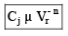n = (1/2)For abrupt or step graded junction

n = (1/3) Linearly graded junction

n = (1/2.5)  Diffused junctions
n = 2 Hyper abrupt junctions

Symbol: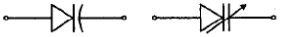Equivalent circuit of varactor diode: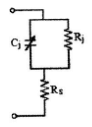Applications of varactor diode:
1. For direct generation of FM
2. Harmonic generation
3. Microwave frequency mulitplication
4. Low noise amplification
5. Pulse generation and pulse shaping

Parametric Amplifier :

• it is amplifier which uses a non-linear reactance or time varying reactance for its amplification.
• Due to its low noise characteristics, it is extensively used in systems such as long range radars and satellite ground stations.

Parametric up-converter

1. fo > fs + fp

f0 - output frequency,,
fp - pump frequency..
fs - signal frequency

2. Power gain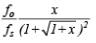Where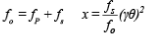g q- Figure of merit for non-linear capacitanc.
Where,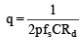Rd - Series resistane of p-n junction diode Noise Figure :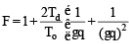Td - Diode temperatuer, TTo - 300º K (room temperature)

Bandwidth :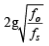Parametric Down Converter:

Power Gain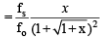f= fp – fs             fi = Idler frequency

Power gain =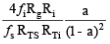Rg - Output resistance of signal generator Ri - Output resistance of idler generator RTS - Total series resistance at fs RTi - Total series resistance at fi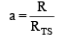where,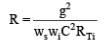Bandwidth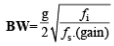Tunnel Diode (Esaki diode): Symbol :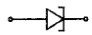•  It is diode which exhibits a negative resistance over a part of the forward bias characteristics.
• Because of the thin junction and short transit time, it is used for microwave frequency switching.

Equivalent circuit: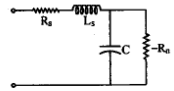I-V Characteristics: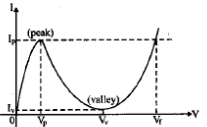•  It is highly doped and have very-vey narrow depletion layer.
• Fabricated with Ga and GaAs. Si is not used because it is difficult to achieve high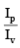with

Resistive cut-off frequency: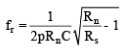Rs - Resistance of packing circuit of tunnel diode
Rn - Negative resistance of tunnel diode
C - Junction capacitance of tunnel diode

Self Resonance frequency: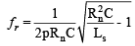Ls - Inductance of pa ckaging circuit of tunnel diode Gain of tunnel diode: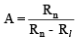• It is used as amplifier and self excited  mixer.
• It is used in high speed switching and logic operations
• it is used for low power oscillators up to 100 GHz, also.

Transferred Electron devices (TEDs): Difference between transistors and TED
1. TEDs have no p-n junctions like transistors.
2. Transistors a re fabricated from Ge or Si, where as TEDs are from GaAs, InP, CdTe.
3. TRansistor s operate with warm electrons where as TEDs operates with hot electrons.

Microwave amplification and oscillations are derived from the bulk negative resistance property of uniform semiconductors rather than from the junction negative resistance property between two different semiconductors as in tunnel diode.

• In negative resistance the current and voltage are out of phase by 180º.

Gunn Effect:

• When a crystal of GaAs is subjected to a high electric field of 2-3 KV/ cm, it has sustained oscillatroy voltage and current across it such that the frequency of produced oscillations depends on the transit time of electron over tha length of crystal.
• As per the effect, the carrier drift velocity linealy incrases from zero to maximum when electric field is varied from zero to threshold value.
• When electric field is beyond 3 KV/cm, the drift velcoty decreases and diode exhibits a negative resistance.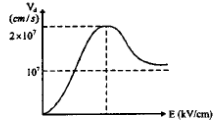• Gunn Effect is properly explained according to Ridley -Watkins-Hilsum theory. According to RWH theory, a negative differential resistance is developed in a bulk of solid state semiconductor compound, when either voltage or current is applied to the terminals of sample.

Gunn diodeL: modes of operation 1 .

Gunn Oscillatory Mode:

For this mode f L  107 cm/s to 2×107 cm/s, 1012/ cm2 < n0L<1014/cm2

Frequency of oscillation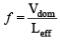Vdom - Domain Velocity Leff - Effective length that the domain travels from the time it is formed until the time that now domain begins to form

• Due to variation of drift velocity with the electric field field there are three possible domain modes for the Gunn oscillation mode.
(a ) Transit time domain mode: (fL  107 cm/sec.)
(b) Delayed domain mode: (106 cm/sec < fL < 2 × 107 cm/sec), Efficiency = 20%
(c) Quenched domain mode: (fL > 2×107 cm/sec), Efficiency = 13%

2 . Limit ed space charge accumulation mode (LSA mode): fL < 2 × 107 cm/sec, n0L  1012/cm2,

Efficiency : 20%

When the frequency is very high the domains do not have sufficient time to form while the field is above threshold. As a result, most of domains are maintained in negative conductance state during a large fraction of the voltage cycle.

3. Stable amplification mode: (n0L < 1012/cm2) In this mode device exhibits amplification at transit time frequency rather than spontaneous oscillation.

Avalanche transit time device:

• This device works on the principle of conduction due to severe breakdown.
• Avalanche diode oscillation uses carrier impact ionization and drift in high field region of a semiconductor junction to produce a negative resistance at microwave frequencies.

• It is n+ – p – i – p+ diode
• The read diode oscillator of n+ – p – i – p+ diode is biased in reverse and mounted in a microwave cavity. The impedance of cavity is mainly inductive and  is matched to mainly cpacitive impedance of the diode to form a resonatnt circuit.
• Transit time of hole across the drift region L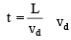vd - Avalanche breakdown voltage

• Avalanche multiplication factor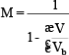V - Applied voltage Vb - Avalanche breakdown voltage n - 3 to 6 for Si

• Read diode supplies a.c. energy, so it has a negative Q factor.
• Frequency of socillation or resonant frequency: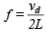L - Drift region Length

• Output power and quality factor a read diode; P = 0.707 VaId W/area Va = mplitude of AC voltage Id = Direct current supplied by Dc bias

2. MPATT  diodes (Impact Ionization Avalanche Transit time diodes):

• A combination of delay involved in generating avalanche current multiplication togeter with delay due to transit time through a drift space, provides 180º phase difference between applied voltage and resulting currrent.
• Three structures of IMPATT diodes:
1. P+ –n – n+ (Abrupt p-n junction)
2. p+ – p – n – n+ (Linearly graded p-n junction)
3. p – i – n

Resonant frequencyVd - Carrier drift velocity L - Drift region length.

Transit time t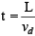Efficiency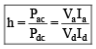Its efficiency is around 30%.

• Biggest problem with IMPATT diode is hsot noise.

So, it is not as good as Klystron or Gunn diodes.

• Its Noise figure is higher than TWT or Gunn diode when it is used as an amplifier.
• IMPATT diodes are most powerful continuous wave solid state microwave power sources.

TRAPATT diodes (Trapped Plasma Avalanche Triggered Transit diodes):

• It is high efficiency microwave generator capable of operating in wide range of frequencies upto several hundred GHz.
• It is either n+ – p – p+ or p+ – n – n+ diode.

Avalanche zone velocity: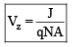J - Current density
N - Doping concentration of n – region

• TRAPATT diode generally exhibit a considerably higher noise figure than IMPATT diode and of upper operating frequency appears to be practically limited to below the millimeter wave region.
• Frequency of TRAPATT diode is limited by the rate of charge of plasma. So it can operate comparatively at lower frequency. Efficiency is 15 to 40%
• Higher Noise figure than IMPATT.

BARITT diode (Barrier Injected Transit Time diode):

• It has long drift regions like IMPATT diode
• It has structures like p – n – p, n – p – v – p p – n – metal and metal – n – metal.
• The diode exhibits a negative resitance for transit angles p and 2p. The optimum transit angle is approximately equal to 1.6 p.
• These diodes are less noisy than IMPATT diodes ad NOise figure is also low.
• Their disadvantage is that they have narrow bandwidth and small power output.

Critical voltage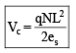N - Dopint concentration L - semiconductor thickness

Breakdown electric field =Breakdown voltage Vbd = 2Vc

The document Microwave Semiconductor Devices Notes | Study Basic Electronics Engineering for SSC JE (Technical) - Electrical Engineering (EE) is a part of the Electrical Engineering (EE) Course Basic Electronics Engineering for SSC JE (Technical).
All you need of Electrical Engineering (EE) at this link: Electrical Engineering (EE)Use Code STAYHOME200 and get INR 200 additional OFF

## Basic Electronics Engineering for SSC JE (Technical)

25 docs|22 tests

Track your progress, build streaks, highlight & save important lessons and more!

,

,

,

,

,

,

,

,

,

,

,

,

,

,

,

,

,

,

,

,

,

;